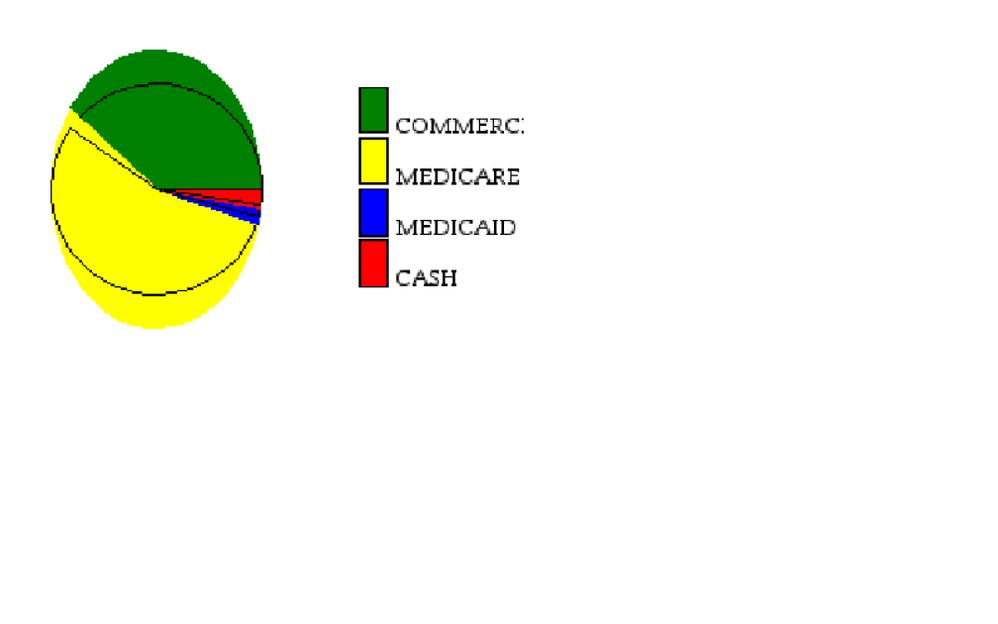## Pie Chart Image Distortion in ODS Layout

Trying to insert a pie chart along with a table from proc report. I am using ODS Layout. My pie chart has distorted colors - it is good in the gchart results window but not in the PDF.

Below is the relevant section for this chart:

ods region x=3.501in y=2in width=3.999in height=1.75in ;

goptions reset=all cback=white hsize=3.5in vsize=1.75in;

proc gchart data=&rxds1(where = (cnt = &i.));

pie lob / sumvar=mat

slice=none

value=none

coutline=BL

matchcolor

other=0

legend=legend1

midpoints="COMMERCIAL" "MEDICARE D"  "MEDICAID" "CASH"

;

legend1 label=none position=(right middle) across=1 shape=bar(3,2);

pattern1 color=green ;

pattern2 color=yellow ;

pattern3 color=blue ;

pattern4 color=red ;

run;

quit;1 ACCEPTED SOLUTION

Accepted Solutions

## Re: Pie Chart Image Distortion in ODS Layout

This is a defect in SAS 9.3 that has been addressed in SAS 9.4.  Unfortunately at this time I do not see a workaround.

4 REPLIES 4

## Re: Pie Chart Image Distortion in ODS Layout

How are you getting it into PDF? You don't show any related code.

## Re: Pie Chart Image Distortion in ODS Layout

Ballardw: thanks for the response - it is part of a longer, but I am adding the related PDF code:

options orientation=portrait papersize=letter nodate nonumber leftmargin=0.5in rightmargin=0.5in topmargin=.001in bottommargin=.001in;

ods escapechar='^';

title;

footnote;

ods pdf file="C:\.......\Output\clyot&i..pdf" notoc;

ods layout start width=8in height=10.9in columns=2;

ods region x=1in y=2in width=2.5in height=1.75in;

Proc Report Data=lob_ord (where = (cnt = &i.)) nowd headline

************

run;

ods region x=3.501in y=2in width=3.999in height=1.75in ;

goptions reset=all cback=white hsize=3.5in vsize=1.75in;

proc gchart data=&rxds1(where = (cnt = &i.));

pie lob / sumvar=mat

slice=none

value=none

coutline=BL

matchcolor

other=0

legend=legend1

midpoints="COMMERCIAL" "MEDICARE D"  "MEDICAID" "CASH"   ;

legend1 label=none position=(right middle) across=1 shape=bar(3,2);

pattern1 color=green ;

pattern2 color=yellow ;

pattern3 color=blue ;

pattern4 color=red ;

run;

quit;

ods region x=1in y=4in width=8in height=6.5in column_span=2;

........

## Re: Pie Chart Image Distortion in ODS Layout

This is a defect in SAS 9.3 that has been addressed in SAS 9.4.  Unfortunately at this time I do not see a workaround.

## Re: Pie Chart Image Distortion in ODS Layout

Thanks for your response Dan. Much appreciated!

Discussion stats
• 4 replies
• 991 views
• 0 likes
• 3 in conversation